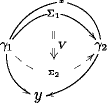# nLab globe

Contents

### Context

#### Higher category theory

higher category theory

# Contents

## Idea

The cellular $n$-globe is the globular analog of the cellular $n$-simplex. It is one of the basic geometric shapes for higher structures.

## Definition

The cellular $n$-globe $G_n$ is the globular set represented by the object $[n]$ in the globe category $G$:

$G_n := Hom_G(-,[n]) \,.$

## Examples

The 0-globe is the singleton set, the category with a single morphism.

The 1-globe is the interval category.

The 3-globe looks like this## Properties

### $n$-Category structure

There is a unique structure of a strict omega-category, an n-category in fact, on the $n$-globe. This makes the collection of $n$-globes arrange themselves into a co-globular $\omega$-category, i.e. a functor

$G \to \omega Cat$
$[n] \mapsto G_n \,.$

### Relation to simplices

The orientals translate between simplices and globes.

See the references at strict omega-category and at oriental.